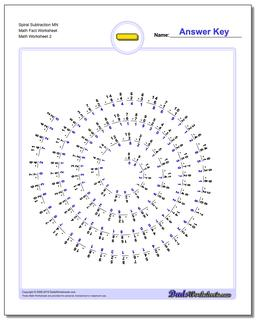PLEASE GO BACK AND USE THE BIG BLUE 'PRINT' BUTTON ON THE PAGE TO PRINT THE WORKSHEET CORRECTLY!Sorry for the trouble! The browser won't print the embedded worksheet PDF directly using the normal 'Print' command in the file menu, so you need to click the big 'Print' button to send just the worksheet and not the surrounding page to the printer.

# Math Worksheets: Subtraction: Subtraction: Spiral Subtraction MN Math Fact Worksheet (Second Worksheet)## Spiral Subtraction MN Math Fact Worksheet (Second Worksheet)

PropertyValue
DescriptionSpiral Subtraction MN Math Fact Worksheet: Tired of the same old math fact worksheets with rows and rows of problems? Time to try something unique. These worksheets present the subtraction facts in a spiral layout that provides fun a twist on memorizing the basic math facts. They use the same fact layouts as the spaceship math sheets above, so try the first two sets worksheets if you are looking for the full set of subtraction facts or practice without the easier problems, or look at the others for an incremental approach to learning everything related to the trusty minus sign! (Second Worksheet)
Resource TypeWorksheet